#Chiba Medical J.　96E：41－46， 2020

doi:10.20776/S03035476-96E-2-P41

[ Original Article ]

## New material property conversion equation for bone strength measurement for CT-based finite element modeling

### Abstract

Computed tomography-based finite element analysis (CT-FEA) has widely been used for the mechanical analysis of osteoporotic bones. To perform CT-FEA, a conversion equation of the materials is used; however, various conversion equations for bone have been reported previously. Therefore, the result depends on which conversion equation is used. New conversion equations must be created because slight variations in the results of the validity verification test using CT-FEA occur, even if various equations are used. This study aimed to identify a more accurate new conversion equation and compare it with past reports. A total of 102 cylindrical cancellous bones (diameter, 10 mm) were collected from nine fresh frozen cadavers. They were thawed at room temperature immediately before the tests were conducted. CT was performed with a calibration phantom, and bone density (g/cm3) was calculated from the obtained Hounsfield unit value using FEA software. The specimens were loaded using a universal testing machine, and yield stress and Young’s modulus were calculated. The correlation between Young’s modulus, yield stress, and bone density obtained from each specimen was investigated, and the bone material property conversion equation was obtained. Previous material property conversion equations were compared with the conversion equation of this study. Young’s modulus and yield stress were correlated with bone density, and the bone material property conversion equation was obtained from the approximate formula. Furthermore, this study’s equation is similar to the Keller vertebra equation. The equation determined in the present study can partly provide a considerable actual bone strength in the CT-FEM calculation compared with the previous studies.

### I．Introduction

Computer simulation using finite element analysis (FEA) is widely applied in the industrial field because it allows prediction without actually destroying the object. Conversely, in living bodies such as bones, unlike industrial products, the characteristics of the materials are not uniform even within the same individual, and analysis is difficult.

Bone vulnerability cannot be accurately evaluated by bone density such as dual-energy X-ray absorptiometry. Recently, computed tomography-based finite element analysis (CT-FEA) has been widely used for the mechanical analysis of osteoporotic bones. CT-FEA is a noninvasive in vivo bone strength measurement method. This method quantitatively obtains bone strength by calculating the three-dimensional bone density distribution from the Hounsfield unit value (HU value) of the quantitative computed tomography and further performing a structural analysis. CT-FEM can reproduce not only the elements of bone density but also the elements of bone quality such as the accumulation of microstructure and fractures. Bone strength consists of two factors, bone density and bone quality, and bone density accounts for almost 70% of bone strength1

Young’s modulus and yield stress are proportional to bone density, and bone density is proportional to the HU value2. Therefore, the areas with a high CT value, which is rendered white on the CT, are considered hard and hard-to-break bones, and the areas with a low CT value, which is rendered black, are considered soft and fragile bones. For patient-specific CT-FEA, the conversion equation of the materials is used. However, various conversion equations for bone have been reported in the past: 3the Keyak 4, Keller all 5, Keller vertebra 5, and Carter 6equations (Table 1). Therefore, the result depends on which conversion equation is used. Furthermore, new conversion equations must be created because slight variations in the results of the validity verification test using CT-FEA occur, even if various equations are used. The purpose of this study was to determine a more accurate new conversion equation and compare it with past reports.

Table. 1 Equations proposed by Keyak, Keller all, Keller vertebra, and Carter.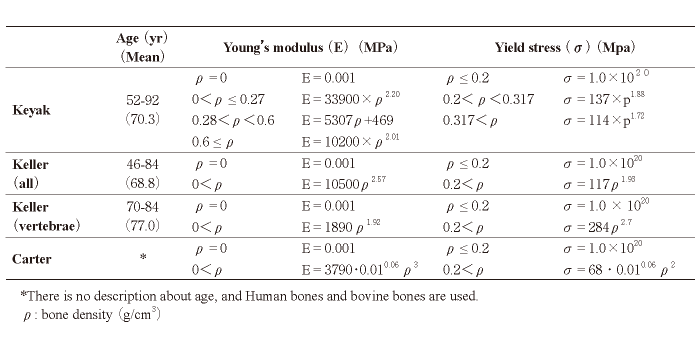### II．Materials and methods

Specimens
We used nine fresh frozen cadavers (four males and five females) from the Clinical Anatomy Laboratory in our university. Additionally, 102 cylindrical cancellous bones (upper extremities, 28; lower extremities, 59; and spines, 25 with a diameter of 10 mm were collected (Fig. 1). The mean age of the cadavers at death was 85.4 years old (range, 78 to 94 years old). The 28 upper limb bones were obtained from the proximal and distal humeri, radii, and ulnae. The 59 lower limb bones were obtained from the proximal and distal femora and tibiae and the calcaneum. The 25 spine bones were obtained from Th10 to L5. All the cadavers were kept at －22℃ and were thawed at room temperature immediately before the tests were conducted. CT was performed using Aquilion ONE (Toshiba Medical Systems, Tokyo, Japan) with the following imaging parameters: 320- row detector; 120 kV; 200 mA; slice thickness, 0.5 mm; and pixel width, 0.3 mm. A calibration phantom (QRM-BDC, QRM, Möhrendorf, DE) containing three hydroxyapatite rods (0 mg/cm3, 100 mg/cm3, and 200 mg/cm3) was tested together with the specimen in water (Fig. 2). Bone density (g/cm3) was calculated from the obtained HU value using FEA software (Mechanical Finder, Research Center for Computational Mechanics, Tokyo, Japan) (Fig. 3)

Mechanical tests
The specimens were loaded using a universal testing machine (Autograph AG-20000N X Plus Precision Universal Tensile Tester; Shimadzu, Kyoto, Japan). Each cylindrical specimen was placed on a testing machine, and a compressive load was applied. After a 2N preload, the specimen was compressed at a speed of 10 mm/min to obtain a force-displacement curve (Fig. 4). The magnitude of the load and displacement were recorded continuously, and mechanical failure occurred at the inflection point of the force-displacement curve (Fig. 5). Using the force-displacement curve, a stress- strain curve was then created to determine the yield stress (Fig. 6). Young’s modulus was calculated from the slope of the approximate expression between 20% and 80% of the yield stress.

Evaluation
The correlation between Young’s modulus, yield stress, and bone density obtained from each specimen was investigated, and the bone material property conversion equation was obtained. Furthermore, the material property conversion equations (Keyak, Keller all, Keller vertebra, and Carter) reported in the past were compared with the conversion equation determined in this study.

The research protocol was in compliance with the Helsinki Declaration; it was approved by Research Ethics Committee of Graduate School of Medicine, Chiba University (Authorization number: #3581). Written informed consent was obtained from all the donors before death.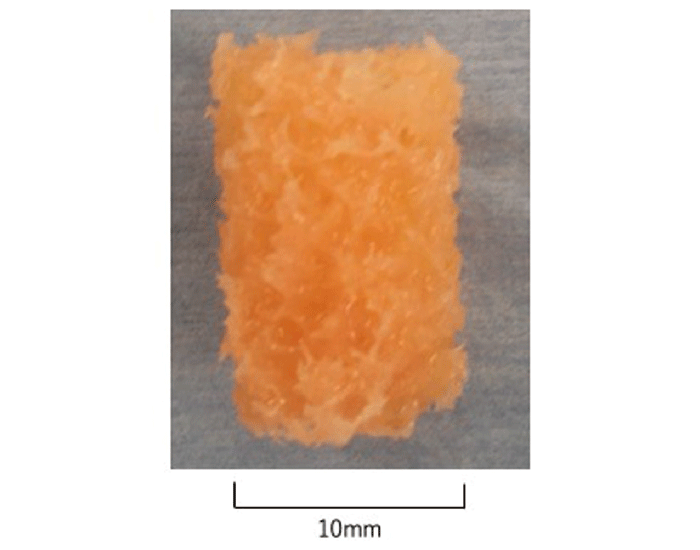Fig.1

A cylindrical cancellous bone with a diameter of 10 mm.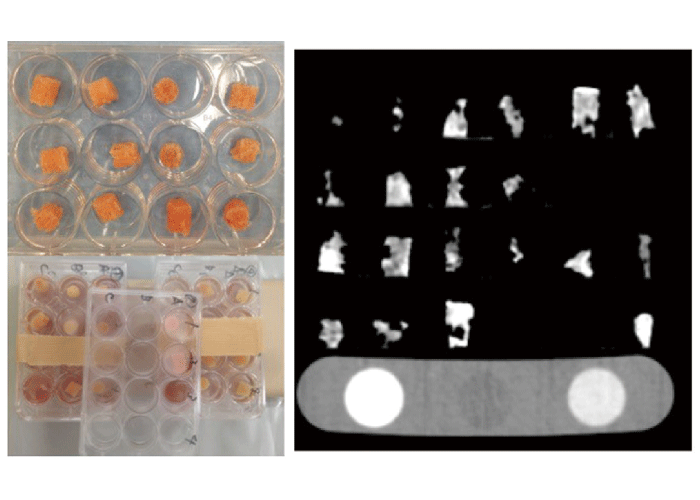Fig.2

CT was performed with the following imaging parameters: 320-row detector; 120 kV; 200 mA; slice thickness, 0.5 mm; and pixel width, 0.3 mm. A calibration phantom containing three hydroxyapatite rods (0 mg/cm3, 100 mg/cm3, and 200 mg/cm3) was tested together with the specimen in water.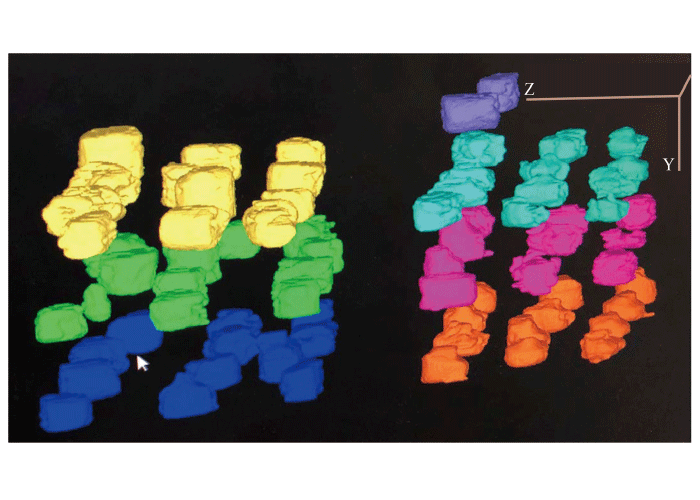Fig.3

A calibration phantom containing three hydroxyapatite rods (0 mg/cm3, 100 mg/cm3, and 200 mg/cm3) was tested together with the specimen in water (Fig.2).
Bone density (g/cm3) was calculated from the obtained CT value using FEA software.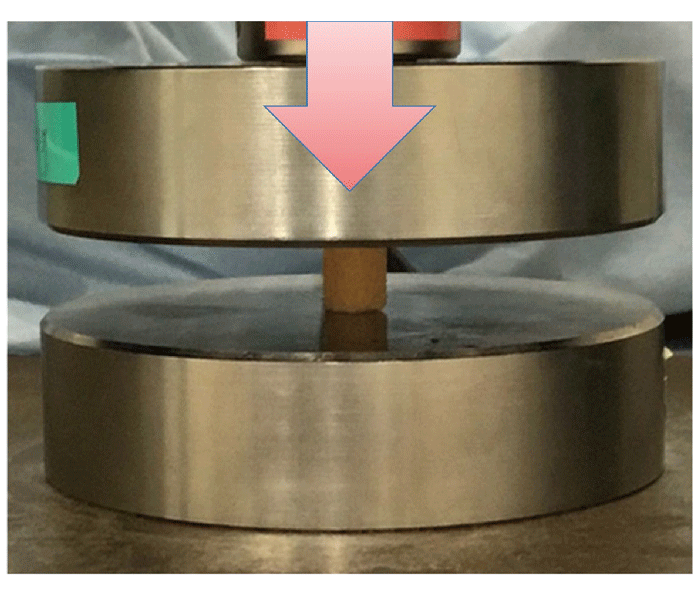Fig.4

Each cylindrical specimen was placed on a testing machine, and a compressive load was applied. After a 2N preload, the specimen was compressed at a speed of 10 mm/min to obtain a force-displacement curve.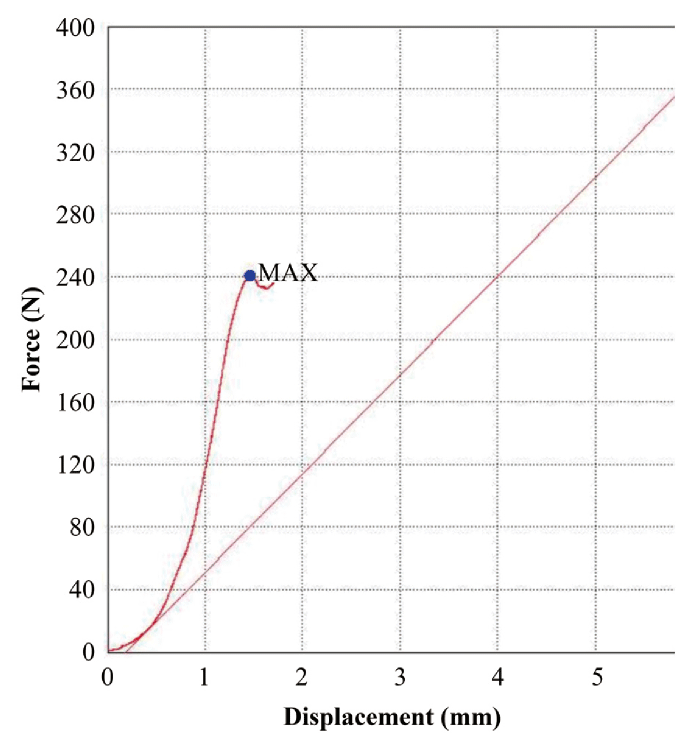Fig.5

The magnitude of the load and displacement were recorded continuously, and mechanical failure occurred at the inflection point of the force-displacement curve.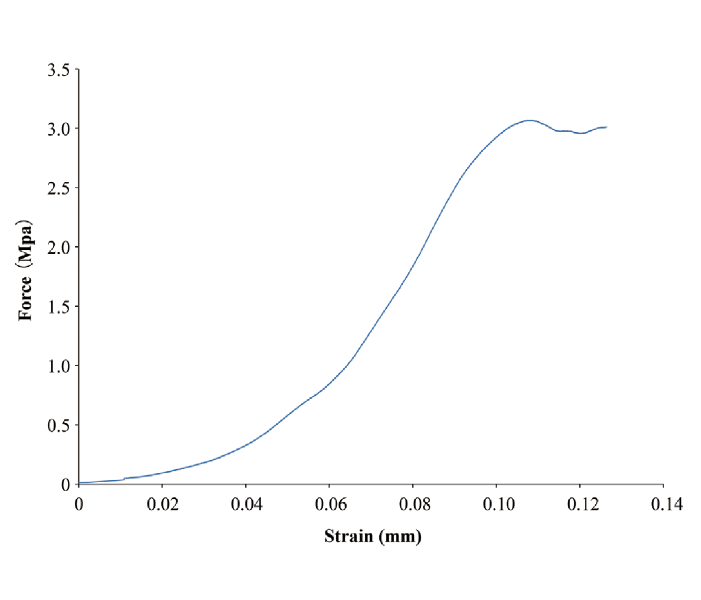Fig.6

Using the force-displacement curve, a stress-strain curve was created to determine the yield stress.

### III．Results

Young’s modulus, as obtained in the mechanical test, is correlated with bone density, and the bone material property conversion equation was obtained from the approximate formula (R2＝0.5422, Y＝1530.6 X1.9213) (Fig. 7). Yield stress is similarly correlated with bone density, and a material property conversion equation was obtained (R2＝0.6049, Y＝116.64 X1.8952) (Fig. 8)

Furthermore, in Young’s modulus, when comparing the equations of Keyak, Keller all, Keller vertebra, and Carter with the equation of this study, our equation was the closest to the Keller vertebra equation (Fig. 9)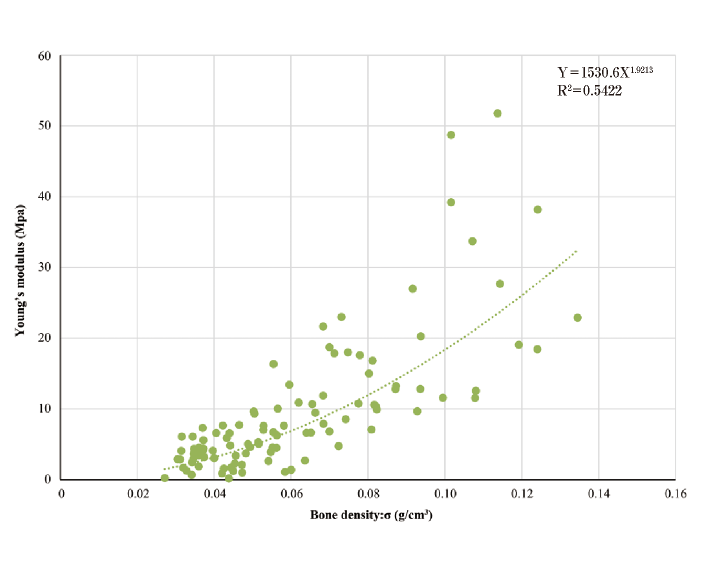Fig.7

Young’s modulus obtained in the mechanical test is correlated with bone density, and the bone material property conversion equation is obtained from the approximate formula (R2 = 0.5422, Y = 1530.6X1.9213)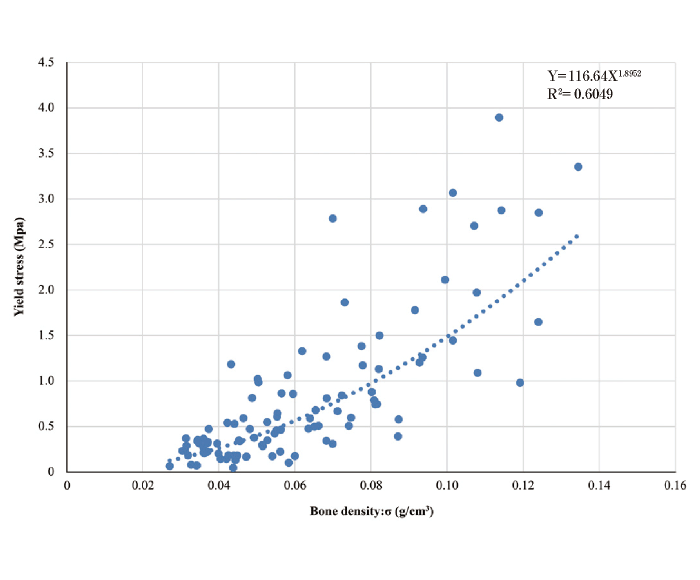Fig.8

Yield stress is similarly correlated with bone density, and a material property conversion equation was obtained (R2 ＝0.6049, Y＝116.64X1.8952).Fig.9

When comparing the Keyak, Keller all, Keller vertebra, and Carter equations with the equation determined in this study for Young’s modulus, this is the closest to the Keller vertebra equation.

### IV．Discussion

Compared with previous reports, the bone material conversion equation obtained in this study is similar to the Keller vertebra equation for Young’s modulus, and it is the most similar to the Keller all equation, followed by the Keller vertebra equation, for yield stress.

Miura et al. reported that the strength of CT-FEM based on the Keller conversion equation is closest to the validity verification test using the fresh frozen cadaver of the proximal femur compared with the Keyak and Carter equations 7. The conversion equation obtained in this study is similar to the Keller vertebra equation, although the coefficients differ (Keller vertebra; 1890, this study; 1530.6); thus, CT-FEM based on this equation is considered to reflect actual bone strength.

Furthermore, Miura et al. also report that CT-FEM using the Keller vertebra conversion equation calculates a Young’s modulus stronger than the actual mechanical test 7. Therefore, the coefficient of this equation is smaller than that of the Keller vertebra equation, so it is expected to be closer to the actual mechanical test.

Keyak tends to have a much higher Young’s modulus than other equations. Keyak conversion equation is widely adopted, but it is reported that the stiffness in CT-FEM is approximately 10 times stronger than the actual mechanical test 7

A limitation of this study is that the average age of the fresh frozen rods used in this experiment was 84 years old and that bones with a particularly high risk of fracture were used. Another limitation is that the compression test was performed as a mechanical test for only cancellous bone and not for cortical bone. Furthermore, the material property conversion equation obtained from this study does not reflect all bone elements of bone strength such as bone collagen quality8

In conclusion, the equation determined in the present study can partly provide a considerable actual bone strength in the CT-FEM calculation compared with the previous studies.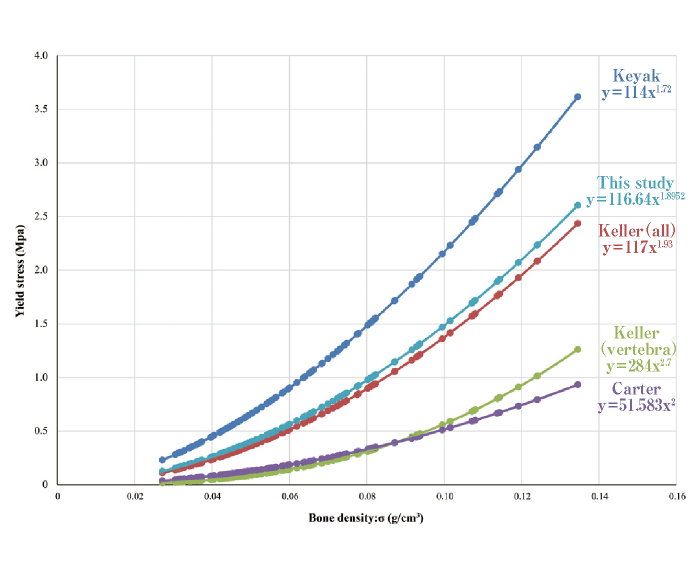Fig.10

When comparing the Keyak, Keller all, Keller vertebra, and Carter equations with the equation determined in this study for yield stress, this is the closest to the Keller all equation, followed by the Keller vertebra equation.

#### Contributors

Guarantor of integrity of the entire study: YM, SO, and TS. Study concepts and design: YM. Experimental studies/data analysis: All authors. Manuscript editing: All authors.

#### Acknowledgments

The co-author, YM, received JSPS KAKENHI Grant Number 16K10886 for this work.

#### Conflict of interest

The other authors have not received any financial support or other benefits from commercial sources for the work.

• 1 ) NIH Consensus Development Panel on Osteoporosis. (2001) Prevention, Diagnosis, and Therapy. Osteoporosis prevention, diagnosis, and therapy. JAMA 285, 785-95.
• 2 ) Les CM, Keyak JH, Stover SM, Taylor KT, Kaneps AJ. (1994) Estimation of material properties in the equine metacarpus with use of quantitative computed tomography. J Ortho Res 12, 822-33.
• 3 ) Keyak JH, Rossi SA, Jones KA, Skinner HB. (1998) Prediction of femoral fracture load using automated finite element modeling. J Biomech 31, 125-33.
• 4 ) Keller TS. (1994) Predicting the compressive mechanical behavior of bone. J Biomech 27, 1159-68.
• 5 ) Carter DR, Hayes WC (. 1977) The compressive behavior of bone as a two-phase porous structure. J Bone Joint Surg Am 59, 954-62.
• 6 ) Helgason B, Perilli E, Schileo E, Taddei F, Brynjólfsson S, Viceconti M (. 2008) Mathematical relationships between bone density and mechanical properties: a literature review. Clin Biomech 23, 135-46.
• 7 ) Miura M, Nakamura J, Matsuura Y, Wako Y, Suzuki T, Hagiwara S, Orita S, Inage K, Kawarai Y, Sugano M, Nawata K, Ohtori S. (2017) Prediction of fracture load and stiffness of the proximal femur by CT-based specimen specificfinite element analysis: cadaveric validation study. BMC Musculoskelet Disord 18, 536.
• 8 ) Saito M, Marumo K. (2010) Collagen cross-links as a determinant of bone quality: a possible explanation for bone fragility in aging, osteoporosis, and diabetes mellitus. Osteoporos Int 21, 195-214.